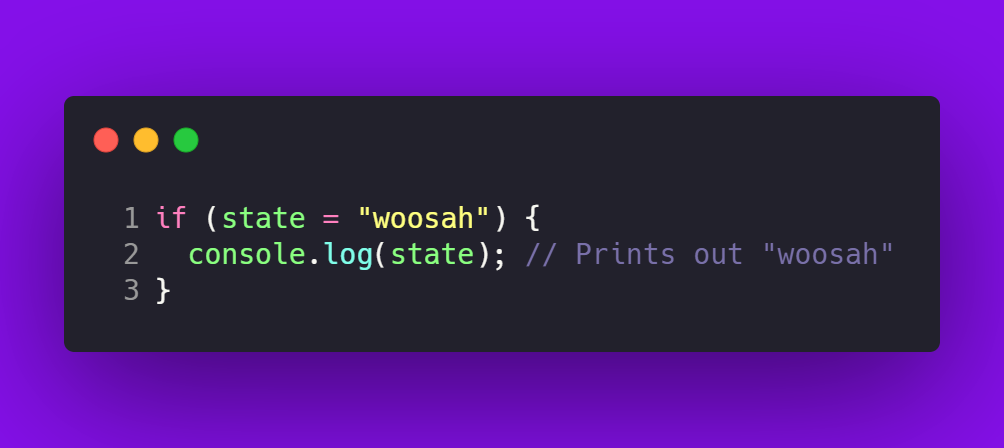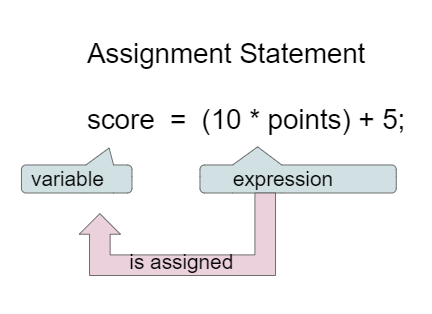## How to declare a variable inside brackets?

Might you have questions like below?

1. js validate and assign online inside if
2. js assign variable inside if bracket
3. is it wrong to declare variables within an if-block?
4. How to declare a variable inside brackets?
5. is it wrong to stick to the ES6 spec and do what I did?

### Basically if-else format like an below codes

```  ```
if (condition) {
var foo = { bar: 'abcd' };
}
```
```

However, JavaScript doesn't have scope blocking. I understand that foo will be null if the condition is not true. Later in the code if I do something like foo.bar I see that the bar cannot be read with an undefined error.

That being said, is it wrong to stick to the ES6 spec and do what I did?

### Playground with examples

##### HTML
``````
<span id="ntrue"></span>
<span id="nfalse"></span>
``````
##### Javascript
``````
// declare Mutable variable
let n = false;
// assign variable as true
if ((n = 2 > 1)) {
// print
document.getElementById("ntrue").textContent = String(n);
}
// assign variable as false
if (!(n = 2 > 4)) {
// print
document.getElementById("nfalse").textContent = String(n);
}
``````

N=(n = 2 > 1)

N=!(n = 2 > 4)

### Example using RegExp

``````const regex = /(?:(?:(\d+):)?(?:(\d+):))?(\d+)(?:\.(\d+))?/gm;

// Alternative syntax using RegExp constructor
// const regex = new RegExp('(?:(?:(\\d+):)?(?:(\\d+):))?(\\d+)(?:\\.(\\d+))?', 'gm')

const str = `1:1.1`;
let m;
// this loop running until regex.exec return null
while ((m = regex.exec(str)) !== null) {
// This is necessary to avoid infinite loops with zero-width matches
if (m.index === regex.lastIndex) {
regex.lastIndex++;
}

// The result can be accessed through the `m`-variable.
m.forEach((match, groupIndex) => {
console.log(`Found match, group \${groupIndex}: \${match}`);
});
}``````

### Example Images## Conclusion

Defining or assigning and validating variable inside if-else scope is allowed, the example like above codes.